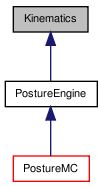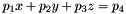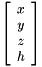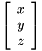# Kinematics Class Reference

Forward and inverse kinematics calculations using Tekkotsu output indices. More...

#include <Kinematics.h>

Inheritance diagram for Kinematics:[legend]

## Detailed Description

Forward and inverse kinematics calculations using Tekkotsu output indices.

You should read the Kinematics tutorial to get a general understanding of the math involved and diagrams for usage with supported robots.

This class involves all aspects of the forward kinematics: calculations concerning locations and orientations in space given a known set of joint configurations. There is a global instantiation of Kinematics named kine, which can be used to perform these calculations regarding the joint positions currently in state.

To perform kinematics on a hypothetical set of joint values, use PostureEngine or one of its subclasses. PostureEngine also adds inverse kinematic functions, which will allow you to determine joint angles in order to reach a given point.

Wherever a reference frame index is requested, you can simply supply one of the output indexes in the usual manner:

 kine->linkToBase(HeadOffset+TiltOffset);


However, there are also a number of points on the body which are not joints, but should have their own reference frames, such as the base frame, or the camera. These frames have their own indices, listed in the robot info file for the model in question (such as ERS7Info.h), with names ending in FrameOffset.

 kine->linkToBase(CameraFrameOffset);


Example code:

  // Find the ray from the camera to whatever the near-field IR is hitting:
fmat::Column<3> camera_ray = T*fmat::pack(0,0,state->sensors[NearIRDistOffset]);
float x; // x will be in the range ±1 for resolution layer independence
float y; // y ranges ±y_dim/x_dim (i.e. ±1/aspectRatio)
config->vision.computePixel(camera_ray,camera_ray,camera_ray,x,y);


Finally, for each model we have created a database of "interest points" -- locations of notable interest on the body of the robot. These may be of use to people attempting to use the limbs to manipulate objects. To access these interest points, call getInterestPoint with the name of the interest point, obtained from the diagrams.

Note that you can pass a comma separated list of interest point names and the result will be the midpoint of those interest points:

 kine->getInterestPoint(BaseFrameOffset,"LowerInnerFrontLFrShin,LowerOuterFrontLFrShin");

PostureEngine for inverse kinematics

Definition at line 68 of file Kinematics.h.

List of all members.

## Classes

struct  InterestPoint
holds the position and attached link of a given interest point More...

## Public Member Functions

Kinematics ()
Constructor, pass the full path to the kinematics configuration file.
Kinematics (const Kinematics &k)
Copy constructor, everything is either update-before-use or static, copy is normal init.
Kinematicsoperator= (const Kinematics &k)
Assignment operator, everything is either update-before-use or static, assignment is no-op.
virtual ~Kinematics ()
Destructor.
const KinematicJointgetRoot ()
Returns a pointer to the root of the kinematic tree.
const KinematicJointgetKinematicJoint (unsigned int idx) const
returns the KinematicJoint structure for the specified Tekkotsu output or reference frame offset
fmat::Column< 3 > getPosition (unsigned int idx) const
Returns a matrix for transforming from link frame j to base frame.
Returns a matrix for transforming from the base frame to link j frame.
fmat::Transform baseToLocal ()
returns a transformation to account for standing pose, where the origin of the "local" space is the projection of the base frame origin along the ground plane normal
fmat::Transform baseToLocal (const PlaneEquation &plane)
returns a transformation to account for standing pose, where the origin of the "local" space is the projection of the base frame origin along the ground plane normal
fmat::Transform localToBase ()
returns a transformation to account for standing pose, where the origin of the "local" space is the projection of the base frame origin along the ground plane normal
fmat::Transform localToBase (const PlaneEquation &plane)
returns a transformation to account for standing pose, where the origin of the "local" space is the projection of the base frame origin along the ground plane normal
void getInterestPoint (const std::string &name, unsigned int &link, fmat::Column< 3 > &ip, bool convertToJoint=false)
Returns the location of a named point and the link it is attached to.
fmat::Column< 3 > getInterestPoint (unsigned int link, const std::string &name)
Returns the location of a named point, relative to any desired reference frame.
void calcLegHeights (const fmat::Column< 3 > &down, float heights[NumLegs])
Calculate the leg heights along a given "down" vector (0 is level with base frame).
LegOrder_t findUnusedLeg (const fmat::Column< 3 > &down)
Find the leg which is in least contact with ground.
PlaneEquation calculateGroundPlane ()
Find the ground plane by fitting a plane to the lowest 3 interest points.
PlaneEquation calculateGroundPlane (const fmat::Column< 3 > &down)
Find the ground plane by fitting a plane to the lowest 3 interest points.
fmat::Column< 4 > projectToPlane (unsigned int j, const fmat::Column< 3 > &r_j, unsigned int b, const PlaneEquation &p_b, unsigned int f, float objCentroidHeight=0)
Find the point of intersection between a ray and a plane.
fmat::Column< 4 > projectToGround (unsigned int j, const fmat::Column< 3 > &r_j, float objCentroidHeight=0)
Find the location of an object on the ground from an arbitrary ray r_j in reference frame j (probably CameraFrameOffset).
fmat::Column< 4 > projectToGround (const VisionObjectEvent &visObj, float objCentroidHeight=0)
Find the location of an object on the ground (the easy way from a vision object event (i.e. EventBase::visObjEGID)).
fmat::Column< 4 > projectToGround (const VisionObjectEvent &visObj, const PlaneEquation &gndPlane, float objCentroidHeight=0)
Find the location of an object on the ground with a custom ground plane specification.
virtual void update () const
refresh the joint settings in root from WorldState::outputs

## Static Public Member Functions

static fmat::Column< 3 > pack (float x, float y, float z)
A simple utility function, converts x,y,z,h to a fmat::Column<3>
static fmat::Column< 4 > pack (float x, float y, float z, float h)
A simple utility function, converts x,y,z,h to a fmat::Column<4>
static void unpack (const fmat::SubVector< 4, const float > &m, float &ox, float &oy, float &oz)
A simple utility function, pulls the first 3 rows of the first column, divides each by the fourth row, and stores into ox, oy, and oz.

## Protected Types

typedef std::map< std::string,
InterestPoint
InterestPointMap
we'll be using the hash_map to store named interest points

## Protected Member Functions

void init ()
Called by constructors to do basic setup - first call will read Config::motion_config::kinematics from disk, future initializes reuse static roconfig.

## Static Protected Member Functions

static void initStatics ()
initializes static variables -- only call if not staticsInited
static void checkStatics ()
checks that statics have been initialized, and calls initStatics if they are missing

## Protected Attributes

KinematicJoint root
the root of the kinematic tree
unsigned int lastUpdateTime
determine if the joints are up to date (compare to WorldState::lastSensorUpdateTime)
KinematicJointjointMaps [NumReferenceFrames]
holds mapping from tekkotsu output index to chain and link indicies

## Static Protected Attributes

static bool staticsInited = false
initially false, set to true after first Kinematics is initialized
static InterestPointMap ips
these interest points are shared by all Kinematics classes (i.e. all PostureEngines)

## Member Typedef Documentation

 typedef std::map Kinematics::InterestPointMap [protected]

we'll be using the hash_map to store named interest points

Definition at line 290 of file Kinematics.h.

## Constructor & Destructor Documentation

 Kinematics::Kinematics ( )

Constructor, pass the full path to the kinematics configuration file.

Definition at line 71 of file Kinematics.h.

 Kinematics::Kinematics ( const Kinematics & k )

Copy constructor, everything is either update-before-use or static, copy is normal init.

Definition at line 76 of file Kinematics.h.

 Kinematics::~Kinematics ( )  [virtual]

Destructor.

Definition at line 40 of file Kinematics.cc.

## Member Function Documentation

Returns a matrix for transforming from the base frame to link j frame.

Parameters:
 [in] link the output offset, see class notes for values

Definition at line 118 of file Kinematics.h.

 fmat::Transform Kinematics::baseToLocal ( const PlaneEquation & plane )

returns a transformation to account for standing pose, where the origin of the "local" space is the projection of the base frame origin along the ground plane normal

Definition at line 44 of file Kinematics.cc.

 fmat::Transform Kinematics::baseToLocal ( )

returns a transformation to account for standing pose, where the origin of the "local" space is the projection of the base frame origin along the ground plane normal

Definition at line 131 of file Kinematics.h.

Referenced by baseToLocal(), Grasper::ReleaseArm::doStart(), and localToBase().

 void Kinematics::calcLegHeights ( const fmat::Column< 3 > & down, float heights[NumLegs] )

Calculate the leg heights along a given "down" vector (0 is level with base frame).

This can be based on either the gravity vector from accelerometer readings, or if that may be unreliable due to being in motion, you could do some basic balance modeling and pass a predicted vector. This uses the interest point database to find the lowest interest point for each leg

Note:
on Aibo platforms, if packing accelerometer readings, don't forget to negate the "left" accelerometer!

Definition at line 115 of file Kinematics.cc.

Referenced by findUnusedLeg().

 PlaneEquation Kinematics::calculateGroundPlane ( const fmat::Column< 3 > & down )

Find the ground plane by fitting a plane to the lowest 3 interest points.

Note:
on Aibo platforms, if packing accelerometer readings, don't forget to negate the "left" accelerometer!
Returns:
vector of the form, relative to the base frame

Definition at line 173 of file Kinematics.cc.

 PlaneEquation Kinematics::calculateGroundPlane ( )

Find the ground plane by fitting a plane to the lowest 3 interest points.

This function merely calls the other version of calculateGroundPlane with the current gravity vector as the "down" vector.

Returns:
vector of the form, relative to the base frame

Definition at line 159 of file Kinematics.cc.

 static void Kinematics::checkStatics ( )  [static, protected]

checks that statics have been initialized, and calls initStatics if they are missing

Definition at line 261 of file Kinematics.h.

Referenced by init().

 LegOrder_t Kinematics::findUnusedLeg ( const fmat::Column< 3 > & down )

Find the leg which is in least contact with ground.

calcLegHeights()
Note:
on Aibo platforms, if packing accelerometer readings, don't forget to negate the "left" accelerometer!
Returns:
index of leg which is highest in reference to gravity vector

Definition at line 147 of file Kinematics.cc.

 fmat::Column< 3 > Kinematics::getInterestPoint ( unsigned int link, const std::string & name )

Returns the location of a named point, relative to any desired reference frame.

Parameters:
 [in] link the desired link reference frame to give results in [in] name the name of the interest point; varies by model, see the diagrams for your model.

You can pass a comma separated list of interest point names and the result will be the midpoint of those IPs.

Definition at line 89 of file Kinematics.cc.

 void Kinematics::getInterestPoint ( const std::string & name, unsigned int & link, fmat::Column< 3 > & ip, bool convertToJoint = false )

Returns the location of a named point and the link it is attached to.

Parameters:
 [in] name the name of the interest point; varies by model, see the diagrams for your model. [out] link on exit, offset of the link, or -1U if not found [out] ip on exit, the requested point, relative to the link frame returned in link [in] convertToJoint if true and name is relative to a "pure" reference frame (i.e. NumOutputs <= link < NumReferenceFrames), then link and ip will be transformed to the parent joint (if it exists)

Definition at line 67 of file Kinematics.cc.

Referenced by getInterestPoint().

 const KinematicJoint* Kinematics::getKinematicJoint ( unsigned int idx ) const
 fmat::Column<3> Kinematics::getPosition ( unsigned int idx ) const

Definition at line 100 of file Kinematics.h.

 const KinematicJoint* Kinematics::getRoot ( )

Returns a pointer to the root of the kinematic tree.

Definition at line 95 of file Kinematics.h.

 void Kinematics::init ( )  [protected]

Called by constructors to do basic setup - first call will read Config::motion_config::kinematics from disk, future initializes reuse static roconfig.

Reimplemented in PostureMC.

Definition at line 23 of file Kinematics.cc.

Referenced by Kinematics().

 void Kinematics::initStatics ( )  [static, protected]

initializes static variables -- only call if not staticsInited

Definition at line 34 of file Kinematics.cc.

Referenced by checkStatics().

Parameters:
 [in] iL the output offset to convert from, see class notes for values [in] oL the output offset to convert to, see class notes for values

Definition at line 123 of file Kinematics.h.

Referenced by getInterestPoint(), IKCalliope::IKCalliope(), and ArmMC::setFingerGap().

 fmat::Transform Kinematics::localToBase ( const PlaneEquation & plane )

returns a transformation to account for standing pose, where the origin of the "local" space is the projection of the base frame origin along the ground plane normal

Definition at line 140 of file Kinematics.h.

Referenced by localToBase().

 fmat::Transform Kinematics::localToBase ( )

returns a transformation to account for standing pose, where the origin of the "local" space is the projection of the base frame origin along the ground plane normal

Definition at line 137 of file Kinematics.h.

 Kinematics& Kinematics::operator= ( const Kinematics & k )

Assignment operator, everything is either update-before-use or static, assignment is no-op.

Definition at line 83 of file Kinematics.h.

 static fmat::Column<4> Kinematics::pack ( float x, float y, float z, float h )  [static]

A simple utility function, converts x,y,z,h to a fmat::Column<4>

Parameters:
 [in] x the value for the first element [in] y the value for the second element [in] z the value for the third element [in] h the value for the fourth element
Returns:Definition at line 242 of file Kinematics.h.

 static fmat::Column<3> Kinematics::pack ( float x, float y, float z )  [static]

A simple utility function, converts x,y,z,h to a fmat::Column<3>

Parameters:
 [in] x the value for the first element [in] y the value for the second element [in] z the value for the third element
Returns:Definition at line 234 of file Kinematics.h.

 fmat::Column< 4 > Kinematics::projectToGround ( const VisionObjectEvent & visObj, const PlaneEquation & gndPlane, float objCentroidHeight = 0 )

Find the location of an object on the ground with a custom ground plane specification.

gndPlane must be specified relative to the base frame, in the form,

projectToPlane() for more control over projection and results

Definition at line 542 of file Kinematics.cc.

 fmat::Column<4> Kinematics::projectToGround ( const VisionObjectEvent & visObj, float objCentroidHeight = 0 )

Find the location of an object on the ground (the easy way from a vision object event (i.e. EventBase::visObjEGID)).

Parameters:
 visObj the vision object to project objCentroidHeight the height of the object's centroid above the ground Uses the default calculateGroundPlane(), otherwise call the other projectToGround() to specify a custom plane.

Definition at line 219 of file Kinematics.h.

 fmat::Column<4> Kinematics::projectToGround ( unsigned int j, const fmat::Column< 3 > & r_j, float objCentroidHeight = 0 )

Find the location of an object on the ground from an arbitrary ray r_j in reference frame j (probably CameraFrameOffset).

Parameters:
 visObj the vision object to project objCentroidHeight the height of the object's centroid above the ground Uses the default calculateGroundPlane(), otherwise call projectToPlane() to specify a custom plane.

Definition at line 211 of file Kinematics.h.

Referenced by projectToGround().

 fmat::Column< 4 > Kinematics::projectToPlane ( unsigned int j, const fmat::Column< 3 > & r_j, unsigned int b, const PlaneEquation & p_b, unsigned int f, float objCentroidHeight = 0 )

Find the point of intersection between a ray and a plane.

Parameters:
 j is the link number the ray is relative to r_j is the ray through the origin of link j b is the link number the plane is relative to (probably BaseFrameOffset) p_b represents the plane to be intersected f is the link number the results should be relative to objCentroidHeight the height of the object's centroid above the ground

p_b should be of the formReturns:
homogeneous coordinate of intersection (may be infinity)

For projecting to the ground plane, one of the specialized projectToGround() functions may be more convenient.

Mathematical implementation:

We'll convert the ray to the plane's reference frame, solve there. We find a point on the ray (ro_b) and the direction of the ray (rv_b). rv_b does not need to be normalized because we're going to find a scaling factor for it, and that factor accounts for current magnitude.

Proof, p=plane normal vector, d=plane displacement, r = ray direction, o = ray offset, x = [x y z] coordinates, t = scaling factorFind distance from the ray offset (ro_b) and the closest point on the plane.

Object height is applied along the plane normal toward the ray origin (we assume the ray source is "above" ground)

Find scaling factor by projecting ray vector (rv_b) onto plane normal.

Intersection point will be rv_b*dist/align + ro_b, but need to watch out for case where align==0 (rv_b and plane are parallel, no intersection)

Definition at line 468 of file Kinematics.cc.

Referenced by projectToGround().

 static void Kinematics::unpack ( const fmat::SubVector< 4, const float > & m, float & ox, float & oy, float & oz )  [static]

A simple utility function, pulls the first 3 rows of the first column, divides each by the fourth row, and stores into ox, oy, and oz.

Parameters:
 [in] m the column to unpack from, applying scaling factor [out] ox set to the first element of m, divided by fourth element [out] oy set to the second element of m, divided by fourth element [out] oz set to the third row element of m, divided by fourth element

Definition at line 249 of file Kinematics.h.

 void Kinematics::update ( ) const [virtual]

refresh the joint settings in root from WorldState::outputs

Reimplemented in PostureEngine.

Definition at line 554 of file Kinematics.cc.

## Member Data Documentation

 Kinematics::InterestPointMap Kinematics::ips [static, protected]

these interest points are shared by all Kinematics classes (i.e. all PostureEngines)

this is to reduce initialization time, but does mean one robot can't do interest point calculations regarding a different model robot...

Definition at line 294 of file Kinematics.h.

Referenced by calcLegHeights(), and calculateGroundPlane().

 KinematicJoint* Kinematics::jointMaps[NumReferenceFrames] [protected]

holds mapping from tekkotsu output index to chain and link indicies

Definition at line 284 of file Kinematics.h.

 unsigned int Kinematics::lastUpdateTime [mutable, protected]

determine if the joints are up to date (compare to WorldState::lastSensorUpdateTime)

Definition at line 281 of file Kinematics.h.

Referenced by update().

 KinematicJoint Kinematics::root [protected]

the root of the kinematic tree

Definition at line 278 of file Kinematics.h.

Referenced by getRoot(), init(), Kinematics(), and operator=().

 bool Kinematics::staticsInited = false [static, protected]

initially false, set to true after first Kinematics is initialized

Definition at line 287 of file Kinematics.h.

Referenced by checkStatics(), and initStatics().

The documentation for this class was generated from the following files:

 Tekkotsu v5.1CVS Generated Mon May 9 04:59:10 2016 by Doxygen 1.6.3